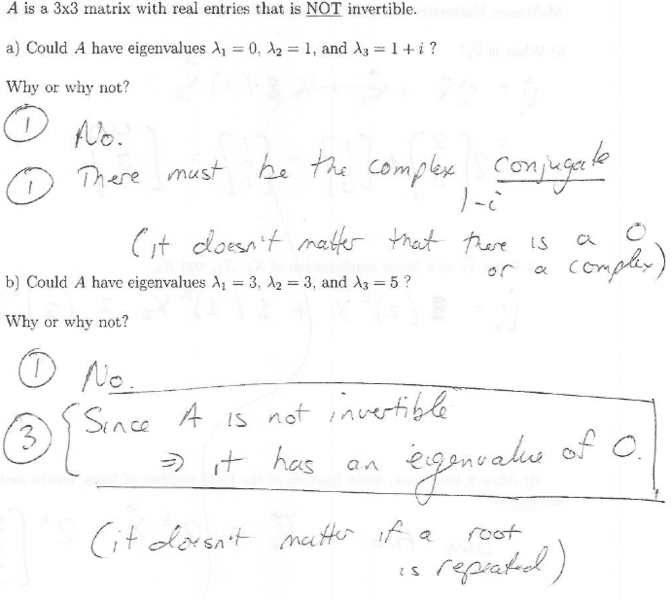# Eigenvalues / eigenvectors concept explaination please

• Ush

#### Ush

Hello
This is a concept question I do not understand. I'm just wondering why the answer is what it is. (the answer is written below the question, I just have no idea where it comes from)#### Attachments

For a, you have to know that complex eigenvalues come in conjugate pairs. That is, if a + bi is an eigenvalue of a matrix A, then so is a - bi. The same goes for eigenvectors. If an eigenvector has entries (a, b + ci) then there is another eigenvector with entries (a, b - ci).

For b, you have to know a couple of things.

A square matrix A is invertible if and only if its column vectors are linearly independent. This is equivalent to saying that a square matrix A is invertible if and only if there are no nontrivial solutions to the equation Ax = 0 (this is because Ax is a linear combination of the column vectors of A).

So then a matrix that is not invertible must have nontrivial solutions to Ax = 0. But in this case, you have found an eigenvector of A with eigenvalue 0. That means every matrix that is not invertible must have eigenvectors corresponding to eigenvalue 0.

Another way to think about it, is that when you are looking for eigenvalues and solving |A - λI| = 0, you are looking for the values of λ that will make A - λI not invertible. But if A is not invertible, then clearly λ = 0 is a solution.

Hope this helps.